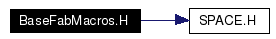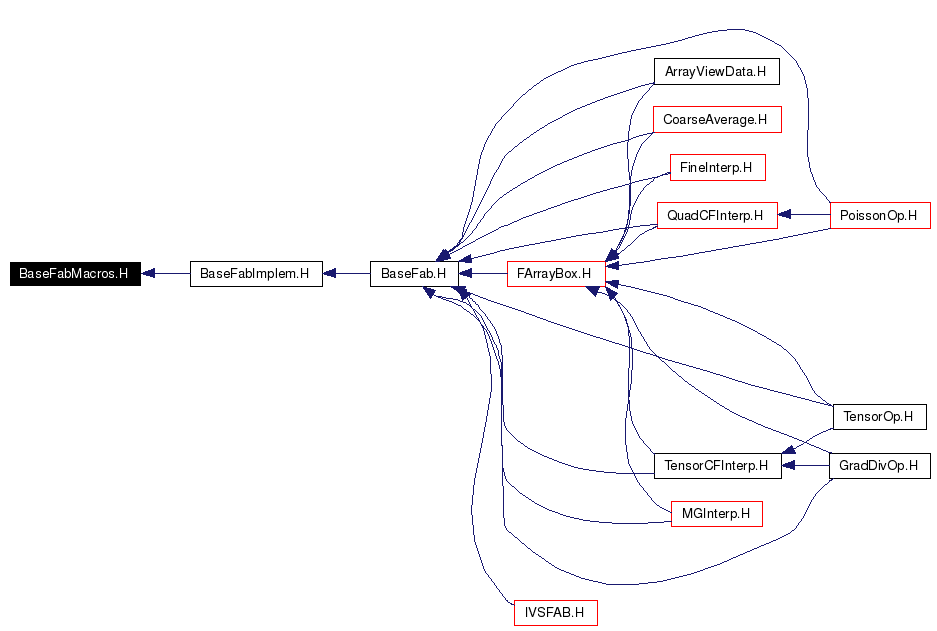# BaseFabMacros.H File Reference

`#include "SPACE.H"`

Include dependency graph for BaseFabMacros.H:This graph shows which files directly or indirectly include this file:Go to the source code of this file.

## Defines

#define ForAllXPencil(T, x)   ForAllXBPencil(T,x,((x).box()),0,((x).nComp()))
#define ForAllX(T, x)   ForAllXBNN(T,x,((x).box()),0,((x).nComp()))
#define ForAllXC(T, x)   ForAllXCBNN(T,x,((x).box()),0,((x).nComp()))
#define ForAllXB(T, x, b)   ForAllXBNN(T,x,(b),0,(x).nComp())
#define ForAllXBC(T, x, b)   ForAllXCBNN(T,x,(b),0,(x).nComp())
#define ForAllThis(T)   ForAllThisBNN(T,this->m_domain,0,this->nComp())
#define ForAllThisC(T)   ForAllThisCBNN(T,this->m_domain,0,this->nComp())
#define ForAllThisB(T, b)   ForAllThisBNN(T,(b),0,this->nComp())
#define ForAllThisCB(T, b)   ForAllThisCBNN(T,(b),0,this->nComp())
#define ForAllThisNN(T, ns, nc)   ForAllThisBNN(T,this->m_domain,ns,nc)
#define ForAllThisXC(T, x)   ForAllThisBNNXC(T,this->m_domain,0,this->nComp(),x,0)

## Define Documentation

 #define ForAllThis ( T ) ForAllThisBNN(T,this->m_domain,0,this->nComp())
 The macro ForAllThis(T) is a shortened form of `ForAllThisBNN' where the Box defaults to the domain of x and the components run over all the components of x.

 #define ForAllThisB ( T, b ) ForAllThisBNN(T,(b),0,this->nComp())
 The macro ForAllThisB(T,b) is a shortened form of `ForAllThisBNN' where the components run over all the components of x.

 #define ForAllThisC ( T ) ForAllThisCBNN(T,this->m_domain,0,this->nComp())
 The macro ForAllThisC(T) is the constant form of ForAllThis(T).

 #define ForAllThisCB ( T, b ) ForAllThisCBNN(T,(b),0,this->nComp())
 The macro ForAllThisCB(T,b) is the constant form of ForAllThisB(T,b).

 #define ForAllThisNN ( T, ns, nc ) ForAllThisBNN(T,this->m_domain,ns,nc)
 The macro ForAllThisNN(T,ns,nc) is a shortened form of `ForAllThisBNN' where the Box defaults to the domain of *this.

 #define ForAllThisXC ( T, x ) ForAllThisBNNXC(T,this->m_domain,0,this->nComp(),x,0)
 The macro ForAllThisXC(T,x) is a shortened form of `ForAllThisBNNXC' where the Box defaults to the domain of *this and the components run over all the components of *this.

 #define ForAllX ( T, x ) ForAllXBNN(T,x,((x).box()),0,((x).nComp()))
 The macro ForAllX(T,x) is a shortened form of `ForAllXBNN' where the Box defaults to the domain of x and the components run over all the components of x.

 #define ForAllXB ( T, x, b ) ForAllXBNN(T,x,(b),0,(x).nComp())
 The macro ForAllXB(T,x,b) is a shortened form of `ForAllXBNN' where the components run over all the components of x.

 #define ForAllXBC ( T, x, b ) ForAllXCBNN(T,x,(b),0,(x).nComp())
 The macro ForAllXBC(T,x,b) is the constant form of ForAllXB(T,x,b).

 #define ForAllXC ( T, x ) ForAllXCBNN(T,x,((x).box()),0,((x).nComp()))
 The macro ForAllXC(T,x) is the constant form of ForAllX(T,x).

 #define ForAllXPencil ( T, x ) ForAllXBPencil(T,x,((x).box()),0,((x).nComp()))
 The Macro ForAllXPencil is a shortened version of ForAllXBPencil where the box is defaulted to the entire domain the the components run over all components of x

Generated on Wed Oct 5 13:53:04 2005 for Chombo&AMRSelfGravity by1.4.1